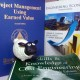# W17_TH_LNG ISOTank Feasibility Study: Best Depreciation Method

##### 1. Problem Evaluation

Continue LNG ISOTank evaluation in blog posting W13, this time author want to research what the best depreciation is. The best mean give more benefit of Author Company such as minimize income tax and also give financial projection of LNG ISOTank operational cash flow.

##### 2. Development of Feasible Alternatives

There are four depreciation models that will compare to get best method:

• Straight Line Method
• Declining Balance Method
• Declining with switchover to Straight Line Method
• MACRS Method
##### 3. Development the Outcome for Each Alternative

Those four method depreciation are choose to compare two group method, commonly use method such as  Straight Line Method and Declining Balance Method, and not commonly use such as Declining with switchover to Straight Line Method and MACRS Method. All method will be compare by depreciation value; bigger depreciation value will get lower income tax.

##### 4. Selection of Criteria

The best method is the method that has the biggest depreciation value. Depreciation value is get from sum present worth of all year depreciation result of each method.

##### 5. Analysis and Comparison of the Alternative

To simulate this depreciation comparison author will use LNG ISOTank 20 feet data. Those data is in table below.

Table 1. LNG ISOTank 20 feet DataNext step is data calculating of each depreciation method, according to straight line method:

dk = (B – SVN) / N

dk* = k. dk for 1 ≤ k ≤ N

BVk = B – dk*

According to Declining Balance method:

d1 = B(R)

dk = B (1 – R)k-1 (R)

dk* = B (1 – (1 – R)k)

BVk = B (1-R)k

Where:

N               = depreciable life of the asset in years

B                = cost basis, including allowable adjustments

Dk             = annual depreciation deduction in year k (1 ≤ k ≤ N)

BVk           = book value at end of year k

SVN          = estimated salvage value at end of year N

dk*             = cumulative depreciation through year k

The calculation results of four depreciation methods are in table below:

Table 2. Straight Line MethodTo demonstrate Declining Balance method, the author use 300% DB equations and result of calculation based on above equation is

Table 3. 300% Declining Balance MethodMethod of Declining Balance Method Switchover to Straight Line Depreciation, in this method the first step is the asset would be depreciated by the 300% DB Method (R =3/N). Because the DB method never reaches a zero BV, suppose that author further specify that at switchover to SL depreciation to will be made to ensure a BV of zero (determined salvage value).

Table 4. DB Method Switchover to SL MethodTo determine the MACRS (GDS) with half year convention assumption and period of analysis in 6 years based on GDS property class.

Table 5. MACRS (GDS) Method, using Half Year ConventionTable 6. PW(14) ComparisonFigure 1. BV Comparison for Selected Methods of Depreciation

##### 6. Alternative Selection

As can be seen above DB Method Switchover to SL Method have the largest PW(14) than other. We can conclude DB Method Switchover to SL Method is the best alternative that more attractive to profitable companies.

##### 7. Performance Monitoring & Post Evaluation Result

To get the best result of method we have to find most appropriate model of depreciation. We should calculate PW of depreciation value of each method, because bigger PW of depreciation value will get lower income tax and more profit of our company.

References

1. Sullivan, G. W. (2014). Engineering Economy 16th Chapter 7 – Depreciation and Income Tax, pp. 332-391
2. Utomo, Dhanu. (2017). W14_UDS_Fuel Tank Truck Feasibility |EMERALD AACE 2017. Retrieved from: http://emeraldaace2017.com/2017/11/21/w14_uds_fuel-tank-truck-feasibility-study-part-2-best-depreciation-method/
3. Planning Planet. (2017). Acquiring Equipment for The Project. Retrieved from http://www.planningplanet.com/guild/gpccar/acquiring-equipment-for-the-project
Posted on

## 1 thought on “W17_TH_LNG ISOTank Feasibility Study: Best Depreciation Method”

1.Dr Paul D Giammalvo says:

Good job Pak Tommy….!!!!!

Almost guaranteed you will see a depreciation problem on your AACE exams and possibly on your Guild certifications as well……

Keep up the good work……

BR,
Dr. PDG, Jakarta